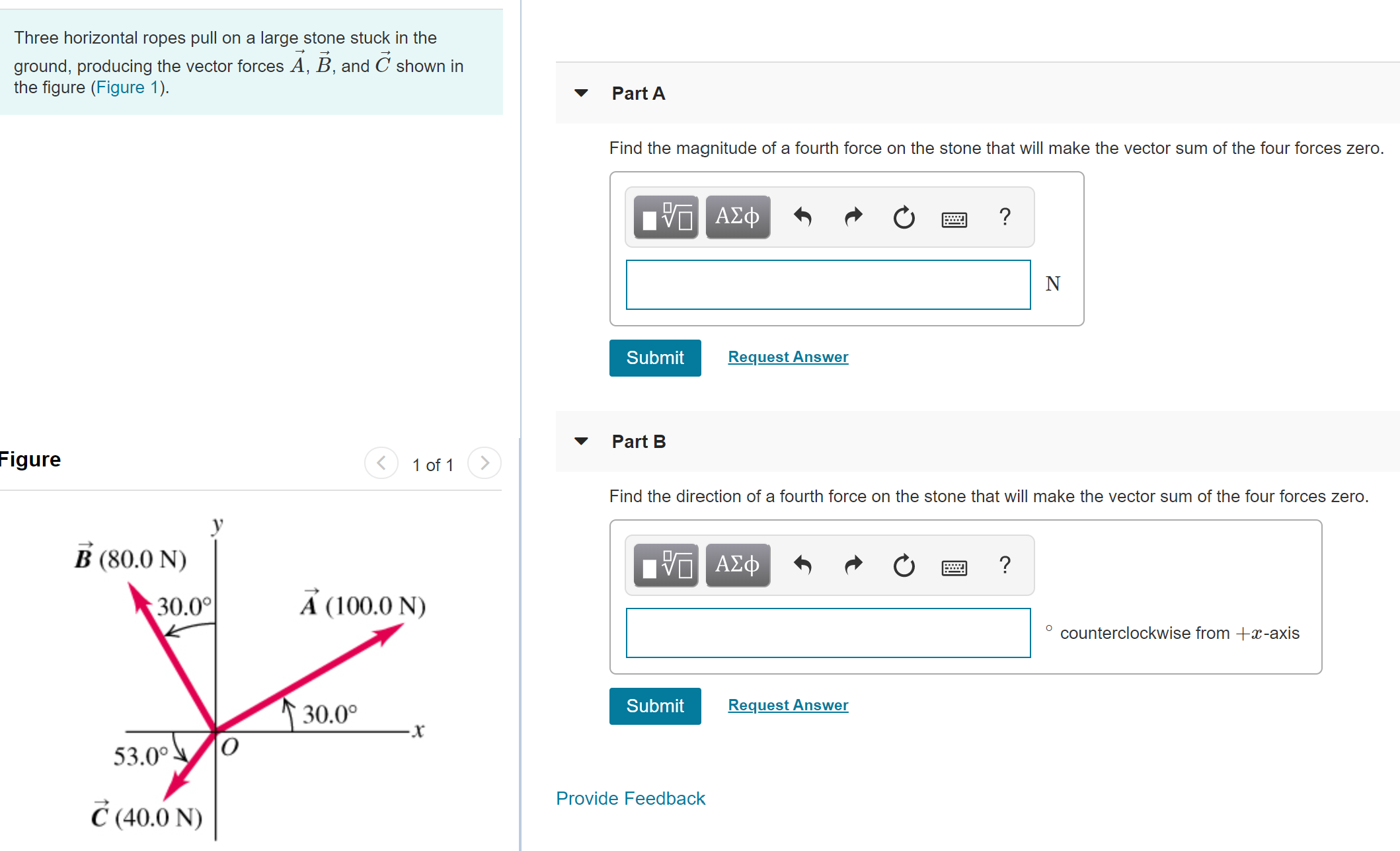# Three horizontal ropes pull on a large stone stuck in theground, producing the vector forces A, B, and C shown inthe figure (Figure 1).Part AFind the magnitude of a fourth force on the stone that will make the vector sum of the four forces zero.να ΑΣφRequest AnswerSubmitPart BFigure1 of 1Find the direction of a fourth force on the stone that will make the vector sum of the four forces zero.B (80.0 N)1ν ΑΣφA (100.0 N)30.0°counterclockwise from +x-axisRequest Answer↑30.0°Submit53.0°Provide FeedbackČ (40.0 N)

Question
13 viewshelp_outlineImage TranscriptioncloseThree horizontal ropes pull on a large stone stuck in the ground, producing the vector forces A, B, and C shown in the figure (Figure 1). Part A Find the magnitude of a fourth force on the stone that will make the vector sum of the four forces zero. να ΑΣφ Request Answer Submit Part B Figure 1 of 1 Find the direction of a fourth force on the stone that will make the vector sum of the four forces zero. B (80.0 N) 1ν ΑΣφ A (100.0 N) 30.0° counterclockwise from +x-axis Request Answer ↑30.0° Submit 53.0° Provide Feedback Č (40.0 N) fullscreen
check_circle

Step 1

(a) The sum of the three forces acting along the horizontal direction is

Step 2

The sum of three forces acting along the vertical direction is

Step 3

The magnitude of a fourth force on the stone that will...

### Want to see the full answer?

See Solution

#### Want to see this answer and more?

Solutions are written by subject experts who are available 24/7. Questions are typically answered within 1 hour.*

See Solution
*Response times may vary by subject and question.
Tagged in

### Vectors and Scalars